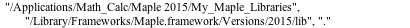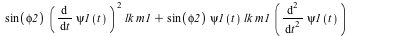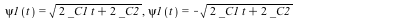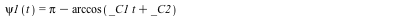## 1506 Reputation

10 years, 249 days

## Covered...

I suggest checking out the autoexecute feature in Maple, which is a per-sheet construct.

Also, there is the .mapleinit file that gets executed upon each restart; this is a global construct.

Seems to me we are pretty well covered here. Pesonally, I use .mapleinit and Moduleload but not the autoexecute sections.

HTH,

M.D.

## First, add "option package" af...

First, add "option package" after the module declaration.

Then, save this as a ".mla" file in a directory in Maple's libname path; like this example:

Here "MathPad" is the name of the module and "MathPad.mla" the filename (they don't have to be identical although I usually keep them like that).

libname is Maple's list of files to look for when searching for packages. It is preset with Maple's package directory and your working directory; I prepend the directory with my own packages to it in the startup file. My libname looks like this:HTH,

Mac Dude

## Clumsily?...

The diffidulty in the given DE is the sin(psi1(t)) that makes it nonlinear. taylor will not expand in terms of psi1(t). Freezing psi1(t) (or just substituting it with a variable) causes taylor to replace essentially 0 because the differential terms now become 0.

I found I had to replace sin(psi1(t)) and cos(psi1(t)) with sin(spsi1) and cos(spsi1) and then do the taylor expansion:

subs(spsi1=psi1(t),convert(taylor(subs(sin(psi1(t))=sin(spsi1),cos(psi1(t))=cos(spsi1),xpr),spsi1,2),polynom));which is not very elegant.

There is however a problem with this: Until you know something about psi1(t) you do not know whether this linearization makes any sense. dsolve will solve the homogeneous linearized DE easily:but it will also solve the original DE:and one now sees that the initial conditions are relevant here in assessing whether this linearization is applicable.

So it isn't quite clear (to me) what you are trying to achieve here...

M.D.

## It has been like this since I started us...

It has been like this since I started using Maple.

The work-around is to "Select all" (Cmd-a on macOS) and then hit the single-step button (!) to run the whole 2nd sheet.

I forget whether Maple will open a new worksheet when another one is running. You may need to open the 2n done first.

M.D.

## You can work around the first problem by...

You can work around the first problem by initially asking for the expansion to 6th order. After that everything appears to work fine; even when the forget(series) statement is removed.

I am not sure about the 2nd issue. The series command makes little sense as it only expands the linear term (x)... what is the point? You are still left with a transcendental equation that has no analytic solution. Maple 2015 hangs on the 2nd try to solve ser for y. Maybe you want expansion by y??

M.D.

## solve...

e1:=0=(1-p)*Ï+Ï*V+Î´*R-(Î¼+Î»+Ï)*S;

e2:=0=p*Ï+Ï*S-(Î¼+Ïµ*Î»+Ï)*V;

e3:=0=Ï*Î»*S+Ï*Ïµ*Î»*V+(1-q)*Î·*i-(Î¼+Î²+Ï)*C;

e4:=0=(1-Ï)*Î»*S+(1-Ï)*Ïµ*Î»*V+Ï*C-(Î¼+Î±+Î·)*i;

e5:=0=Î²*C+q*Î·*i-(Î¼+Î´)*R;

solve([e1,e2,e3,e4,e5],[S,V,C,R,i]);

The result is too messy to print here, see the worksheet. Do note that I is Maple's imaginary unit so I changed I to i.

M.D.

mprimes.mw

## It depends...

It depends on the specifics of your data and what you are trying to achieve. Technically, your Fourier analysis constitutes the test of a hypothesis, the hypothesis being that your samples are taken from a distribution given by your function, the Fourier series. If x could never be negative for fundamental reasons then I'd argue both Fourier series are equally valid; your data cannot discriminate between the two. The catch is that it is hard to imagine a case where x is fundamentally positive and a Fourier series is a valid representation of what is going on. When frequency analysis is meaningful, the independent variable (x) usually is a location or a time variable that is unbound in either direction.

What you really should do is have a model of the underlying distribution, i.e. of the process that generated the data in the first place. If that is symmetric about 0 then you are in a good place and can use the symmetry.

If you need to known the spectral composition of the Fourier series then you need to tread carefully here. Your two series have a significantly different spectrum; if you do not have enough data to discriminate between the two you may not be able to determine the spectrum of the underlying distribution.

M.D.

## elementwise...

About the simplest way is to use the element-wise operations:

B:=sqrt~(1/~A); # note the tildes

More flexible is map(sqrt,A) which maps the sqrt function over all elements of A. RTFM for these. While map and ~ seem to be the same there are somewhat subtle differences, but for this simple case they should produce the same answer.

If the 0 elements in A bother you you can define a new  function

f:=x -> `if`(x>0,sqrt(1/x),0);

and use f~.

Mac Dude

Edit: Ninja'd by Rouben. I like Rouben's way of using an unnamed function...

## It depends......

It isn't quite clear what you want. If it is Maple code you want to compare then you can e.g. write the code out as a .mpl file (which is ASCII) and then either use diff on them (Linux or Mac OS X) or read these back into Maple and use one of the StringTools commands to compare.

The worksheets are xml files, so your comparison of these may yield differences in the xml code as opposed to real code differences.

RTFM on write, read and StringTools to figure out how to use these.

Mac Dude

## Consider a package...

Consider putting the whole Module with all its procs etc. into a text file with extension .mpl. Add "option package" to the module.

You can then make a package suitable for loading using with(); by doindg the following in a small Maple worksheet:

read("/Applications/Math_Calc/Maple 2015/Packages/Lattice/Lattice4.mpl"); # this is one of mine; put your above .mpl file in here with the full path.

LibraryTools:-Save(Lattice,cat(libname,"/Lattice.mla")); # Replace the first "Lattice" with your module name and name the .mla file what you want.

For this to work pre-pend libname with the directory where you want your package to reside. I do that in the startup file for Maple. You do need to preserve what is already in libname else Maple can't find its own modules anymore.

I use Emacs with Joe Riel's maplev mode to edit the .mpl file. Much more convenient than editing in Maple. To update the .mla file just run the small worksheet I suggested above.

M.D.

## Here is another one...

You already have answers, but since with earlier versions of Maple I ran into exactly the same issue as you did, here is how I used to do it. I did find out later, though, that the Mersenne twister used is rather slow.

#----------------------------------------------------------
(* Procedure Frand(rnge)
Returns a random float uniformly distributed across range.
Parameters:   rnge  xmin..xmax the interval
*)
Frand:=proc(rnge)
if (type(rnge,'range')) then
RandomTools[MersenneTwister]:-GenerateFloat64()*(op(2,rnge)-op(1,rnge))+op(1,rnge)
else
RandomTools[MersenneTwister]:-GenerateFloat64()*rnge
end if

end proc;

#------

M.D.

## locals, namespaces and OOP...

Preben already answered the basic question how Maple keeps track of local variables: by their address. Other than that, the x are in fact indistinguishable. Except that, if you e.g. try to subs(x=<something>,...) the subs will silently fail and not do anything visible. Made me tear my hair out when I first ran into this situation (due to an unrelated mistake).

The reason why this happens is that you returned an unassigned local variable, which just returns the variable itself. It isn't quite clear to me why you would want to do that, but of course there maybe perfectly valid reasons I just haven't encountered yet. The fact that you cannot do anything with this variable makes some sense: it is procedure-local and not supposed to be visible outside of the proc, much less changeable. It is a bit confusing, however, as no distinction is made.

But what if you actually want to get access to the x? One way to achieve that is to wrap the whole proc in a module and then have x be an export of the module. Like this:

bar:=module()
export x,foo;
foo:=proc(n);
#local x; # turn this off
x^n;
end proc;
end module;

Run the proc (I use the long form here):

x:=99;
bar:-foo(2);
x := 99
2
x

and you can access both xes:

x;
bar:-x;
99
x

If you load module bar using with(bar) [you may need to use option package to do this] then x will implicitly refer to bar:-x and you need to say :-x to get at the x you assigned 99 to [assuming you assign to x first and then use with(bar)]. In this case you have created a new namespace (bar:-) that can be used to keep variables apart.

Finally, in this case repeated calls to foo will not create new instances of x, naturally, as x belongs to module bar. If you want to create several instances of bar you need to use the copy() function; if you assign bar to two different variables you just get two pointers to the same instance.

Obviously now we are on our way to do "OOP lite" in Maple, a feature introduced in Maple 15 that I quite like and use often in my larger packages.

Thanks for the question/post; it exposed an interesting peculiaroty of Maple.

M.D.

## @Adam Ledger  I am not sure I fully...

@Adam Ledger  I am not sure I fully understand your approach, but I sure have experienced sluggish execution of programs in Maple. ROT13 is a simple algorithm and should not be sluggish. I'd be tempted to implement ROT13 in a table lookup; tables are Maple's associative arrays so you ca use each letter as an index into the table that has the shifted letters. That kind of lookup is quite fast.

If you do numerics, a few hints that may help the situation: First, Maple has the propensity to build huge algebraic expressions if it encounters variables ("names") that are unassigned. The way to detect and/or prevent that is to use evalf often and in case of arrays or vectors to make sure they are declared with "datatype=hfloat". Then, if what is assigned to them isn't a float, it barfs and you can fix the code. You can try evalhf as well but I have run into trouble with it; it is finicky in what can be in its arglist.

It makes sense to evalf an expression before using it in a loop. Evalf will at least simplify any function it can evaluate to a float & in that sense simplify the expression. Sometimes simplify and evalf are needed together to make things faster.

Then there is Compiler:-Compile. I have had relatively little luck with it but you may have better luck. The idea is that you can have a compiled code section which will execute faster. Please read the help on it; I am not an expert in Maple's Compiler operations and there are a number of restrictions.

If you have complicated procs and/or subprocs & the like, the CodeTools package has some tools that will help you to identify the bottleneck in your code. Excessive memory usage and faster than linear growth in executing time with the number of data points (or iterations of a loop) are signs that Maple is trying to do algebraics where you do not want it (even if the final result may be ok).

Cheers,

M.D.

## I don't think so. Depending on the ...

I don't think so.

Depending on the code to be translated I'd consider using a good editor (Emacs, for example) to make he necessary changes "en masse" using e.g. replace-string. This will work for code snippets.

You can call external code from Maple. Look for examples,external Calling in help. I have never used this.

M.D.

## In number 1, you use a dot (.) for multi...

In number 1, you use a dot (.) for multiplication. The dot stands in Maple for non-commutative multiplication. I am not sure what this means in this context but when you replace the dot by an asterisk (*) th eintegral evaluates as expected.

In number 8 a multiplication sign is missing, making Maple think the first x is a function.

Results (Maple 2015):

int(sqrt(x^3*(ax+b)), x);

(1/2)
2   / 3         \
- x \x  (ax + b)/
5

int(sqrt(x*(ax+b)), x);

2               (1/2)
- x (x (ax + b))
3

Mac Dude.

Edit: Forgot your attached file. That one fails because c and v together form a variable cv, which has nothing to do with c*v as I assume you intended.

Result:

int(1/(g-c*v/m), v);
=
/    c v\
ln|g - ---| m
\     m /
- -------------
c

Moral: Use 1-d input and not 2-D input, esp. when you are new to Maple & don't need the typesetting. In prefs, on the Display tab change the Input display to Maple Notation. While you are at it, on the Interface tab change the Default format for new sheets to Worksheet.

﻿